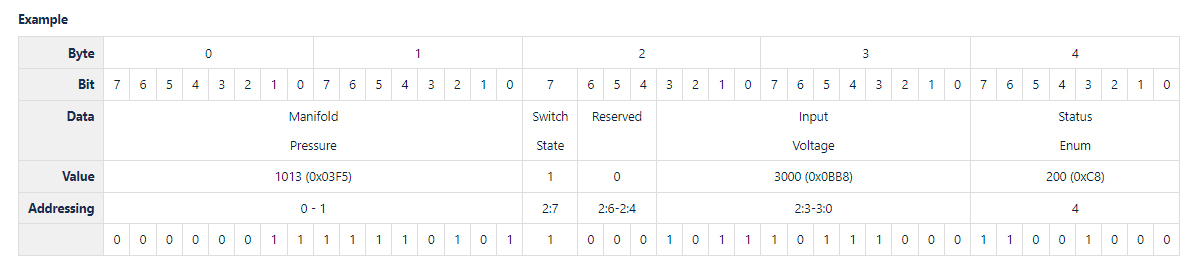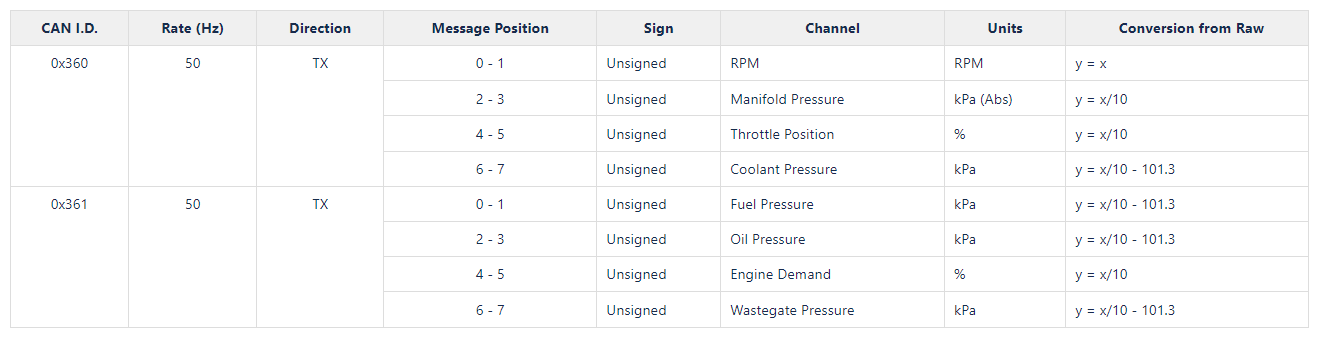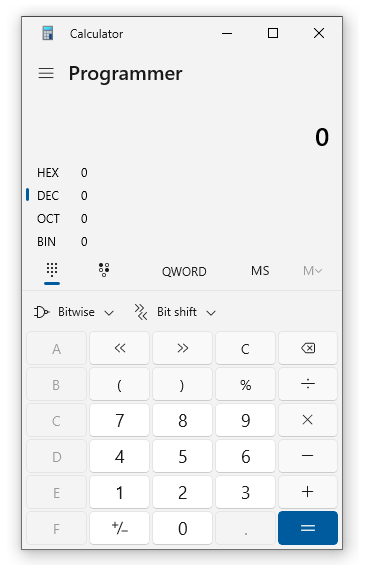# Haltech CAN Protocol Specification

## Key Information

The Haltech CAN bus operates at 1 MBit/s and uses 11-bit IDs, which are expressed in Hexadecimal.

The first byte in a packet is considered byte 0, and the 8th byte is byte 7. The most significant bit in a byte is considered bit 7 and the least significant bit is bit 0.

Data is encoded as big-endian.

Data that is the size of an individual byte (that is byte aligned) are represented with the byte number, e.g., `4` for data at byte 4

Data that crosses multiple bytes (that is byte aligned) are represented with a byte range. The range is inclusive, e.g., `0 - 1` for 2 bytes of data in bytes 0 and 1, `4 - 7` for 4 bytes of data in bytes 4, 5, 6, and 7.

To address data stored in individual bits within a byte, the following notation is used X:Y. The X is the byte number and the Y is the bit number, e.g. `4:0` for bit 0 in byte 4.

To address data stored across many bits that may span bytes the above notation is used but expressed as a range. The range is inclusive, e.g., `6:3 - 7:0` for 12 bits of data starting at bit 3 on byte 6 to bit 0 on byte 7.### Units

The "Conversion from Raw" column in the example protocol specification table below, shows how to convert the raw value in the message to a value known unit as indicated by the "Units" column.

The x symbol represents the raw value and y represents the value converted.

E.g. The "Throttle Position" channel has a raw value of 456. The conversion for it is y = x / 10. Therefore the converted value of y equals:

y = 456 / 10 = 45.6%

If other units are required, it is the responsibility of the device reading these values to perform these conversions. All pressures are absolute, and it is necessary to subtract 101.3 kPa from the final result if gauge pressure is desired.## CAN Units

 Symbol Name Quantity Notes RPM Revolutions per minute Rotational velocity Typically, engine crankshaft rotational speed. kPa kiloPascals - gauge Pressure 0 kPa = standard atmospheric pressure. -101.3 kPa = absolute vacuum. kPa (abs) kiloPascals - absolute Pressure 0 kPa (abs) = absolute vacuum. 101.3 kPa(abs) = standard atmospheric pressure. % Percent ° Degree Angle 360 degrees make up one revolution. Note that a 4-stroke engine cycle requires 2 crankshaft revolutions, so the engine cycle is 720 degrees. lambda 1 lambda = the stoichiometric ratio of the fuel being used.  To convert to typical gasoline/petrol AFR: multiply by 14.7 To convert to ethanol AFR: multiply by 9 To convert to methanol AFR: multiply by 6.47 K Kelvin Temperature A unit of temperature where 0 is absolute zero temperature. It uses the same scale as degrees Celsius. So, an increase of 1K is an increase of 1°C. To convert from Kelvin to degrees Celsius, simply subtract 273.15. Temp_C = Temp_K - 273.15 To convert from Kelvin to degrees Fahrenheit: Temp_F = (Temp_K - 273.15) × 9/5 + 32 Water freezes at 273.15K and boils at 373.15K. km/h kilometers per hour Velocity A common metric speed measurement.  To convert to meters per second: divide by 3.6. To convert to miles per hour: divide by 1.609. m/s2 m/s² meters per second-squared Acceleration A common metric acceleration measurement.  To convert to G: divide by 9.8 dB Decibel cc/min cubic centimeters per minute Volume Flow Rate A volume flow-rate measurement commonly used for fuel injectors.  A common approximate conversion to pounds per hour (lbs/hr) is to cc cubic centimeter Volume 0.001 L. To convert to fluid ounces (fl. oz.): divide by 29.574. L liter Volume Volume. 1000 cc.  To convert to US gallons: divide by 3.785 V Volt ppm parts per million Concentration / Ratio A mass ratio used for representing concentrations of a substance within another. 1,000,000 ppm is a pure substance. E.g., seawater has a salinity of roughly 35,000 ppm, equivalent to 35 grams of salt per kilogram of water. g/m3 g/m³ grams per cubic meter Density Water has a density of 1 million g/m³ ms millisecond Time 1/1000 of a second. s second Time 1/60 of a minute, 1/3600 of an hour. min minute Time 60 seconds. 1/60 of an hour. h hr hour Time 60 minutes. 3600 seconds.

## Bit Field Interpretation

Bit fields are a useful way of indicating the state of multiple things simultaneously within one value.

Typically, each binary digit (bit) represents whether a thing is 0 or 1. 0 typically means false or off, and 1 typically means true or on.

E.g., The Haltech ECU channel "Engine Limiting Method" has 1 as Fuel and 2 as Ignition. This is 0b01 for fuel and 0b10 for Ignition. If both Fuel and Ignition are being used, the two values are added together, giving a value of 1 + 2 = 3.

Decoding bit fields:

####• Select Programmer mode by clicking on the three lines, then Programmer.
• Click the DEC to put it into decimal mode (normal numbers).
• The binary version of your number will be shown next to BIN. The right-most digit has value 1. The next has value 2, then 4, then 8, then 16, etc., that is, doubling each time.

#### b.) Manually decoding bit fields

• To decode a bit-field, look for the largest raw value which is less than or equal to the value you're trying to decode. In this example, 2 is the highest when decoding 3.
• So we know that Ignition is one of the values. Now we need to find the other value(s).
• We simply subtract the 2, and repeat the process until we are left with 0.
• So 3 - 2 = 1. 1 is the highest, so Fuel is one of the values.
• When we subtract the 1 for fuel, we get 1 - 1 = 0. Because we get 0, we are finished decoding the value, and know that both Fuel and Ignition are true.

• # Related Articles

• ## Haltech PD-16 CAN Protocol

Multiplexing We're sending and receiving a lot of data. We'll multiplex our CAN messages to consume as few CAN IDs as possible. If a message is multiplexed then the first byte will be a multiplex ID used to identify the payload. The Multiplex ID has ...
• ## Haltech ECU CAN Broadcast Protocol

Device Version for Full Support* Nexus Series ECU 1.24 Elite Series ECU 3.10 IC7 Dash ? * Earlier versions support parts of this protocol. The most up-to-date ECU and dash firmware available will support most signals. CAN I.D. Rate (Hz) Direction ...
• ## Haltech ECU Comparison Table

STILL UNSURE? If you’ve narrowed your choice down to a couple of ECUs but want to confirm you’ve made the right decision contact our Tech Support Hotline. Remember, our goal is not to just sell you any ECU, we want to provide you with an ECU that’s ...
• ## Haltech CAN System

Haltech CAN System This node allows any CAN Devices to be enabled and for a DTC Severity level to be set for each device. Displays If you have a Haltech CAN Supported Dash it will need to be enabled in this page by clicking the Tick box for the: ...
• ## Haltech Can Bus Selection

The Haltech CAN Bus is used to communicate with all Haltech CAN based expansion devices such as wideband controllers, display dashes and I/O expander boxes. Select the port where you would like the Bus to be broadcast.